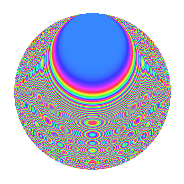# Properties

 Label 945.2.caLevel 945 Weight 2 Character orbit ca Rep. character $$\chi_{945}(368,\cdot)$$ Character field $$\Q(\zeta_{12})$$ Dimension 176 Newforms 1 Sturm bound 288 Trace bound 0

# Related objects

## Defining parameters

 Level: $$N$$ = $$945 = 3^{3} \cdot 5 \cdot 7$$ Weight: $$k$$ = $$2$$ Character orbit: $$[\chi]$$ = 945.ca (of order $$12$$ and degree $$4$$) Character conductor: $$\operatorname{cond}(\chi)$$ = $$315$$ Character field: $$\Q(\zeta_{12})$$ Newforms: $$1$$ Sturm bound: $$288$$ Trace bound: $$0$$

## Dimensions

The following table gives the dimensions of various subspaces of $$M_{2}(945, [\chi])$$.

Total New Old
Modular forms 624 208 416
Cusp forms 528 176 352
Eisenstein series 96 32 64

## Trace form

 $$176q$$ $$\mathstrut +\mathstrut 6q^{2}$$ $$\mathstrut -\mathstrut 2q^{7}$$ $$\mathstrut +\mathstrut O(q^{10})$$ $$176q$$ $$\mathstrut +\mathstrut 6q^{2}$$ $$\mathstrut -\mathstrut 2q^{7}$$ $$\mathstrut -\mathstrut 4q^{10}$$ $$\mathstrut -\mathstrut 4q^{13}$$ $$\mathstrut +\mathstrut 68q^{16}$$ $$\mathstrut +\mathstrut 18q^{17}$$ $$\mathstrut +\mathstrut 12q^{20}$$ $$\mathstrut +\mathstrut 4q^{22}$$ $$\mathstrut -\mathstrut 4q^{25}$$ $$\mathstrut -\mathstrut 4q^{28}$$ $$\mathstrut +\mathstrut 4q^{31}$$ $$\mathstrut +\mathstrut 90q^{32}$$ $$\mathstrut -\mathstrut 4q^{37}$$ $$\mathstrut -\mathstrut 36q^{40}$$ $$\mathstrut +\mathstrut 36q^{41}$$ $$\mathstrut -\mathstrut 4q^{43}$$ $$\mathstrut +\mathstrut 4q^{46}$$ $$\mathstrut +\mathstrut 6q^{47}$$ $$\mathstrut -\mathstrut 36q^{50}$$ $$\mathstrut -\mathstrut 52q^{52}$$ $$\mathstrut +\mathstrut 4q^{55}$$ $$\mathstrut +\mathstrut 96q^{56}$$ $$\mathstrut -\mathstrut 12q^{58}$$ $$\mathstrut -\mathstrut 8q^{61}$$ $$\mathstrut +\mathstrut 78q^{65}$$ $$\mathstrut +\mathstrut 2q^{67}$$ $$\mathstrut -\mathstrut 42q^{70}$$ $$\mathstrut -\mathstrut 4q^{73}$$ $$\mathstrut -\mathstrut 24q^{76}$$ $$\mathstrut -\mathstrut 42q^{77}$$ $$\mathstrut -\mathstrut 36q^{80}$$ $$\mathstrut -\mathstrut 8q^{82}$$ $$\mathstrut +\mathstrut 12q^{83}$$ $$\mathstrut -\mathstrut 4q^{85}$$ $$\mathstrut +\mathstrut 12q^{88}$$ $$\mathstrut -\mathstrut 16q^{91}$$ $$\mathstrut -\mathstrut 72q^{92}$$ $$\mathstrut +\mathstrut 66q^{95}$$ $$\mathstrut -\mathstrut 4q^{97}$$ $$\mathstrut -\mathstrut 12q^{98}$$ $$\mathstrut +\mathstrut O(q^{100})$$

## Decomposition of $$S_{2}^{\mathrm{new}}(945, [\chi])$$ into irreducible Hecke orbits

Label Dim. $$A$$ Field CM Traces $q$-expansion
$$a_2$$ $$a_3$$ $$a_5$$ $$a_7$$
945.2.ca.a $$176$$ $$7.546$$ None $$6$$ $$0$$ $$0$$ $$-2$$

## Decomposition of $$S_{2}^{\mathrm{old}}(945, [\chi])$$ into lower level spaces

$$S_{2}^{\mathrm{old}}(945, [\chi]) \cong$$ $$S_{2}^{\mathrm{new}}(315, [\chi])$$$$^{\oplus 2}$$